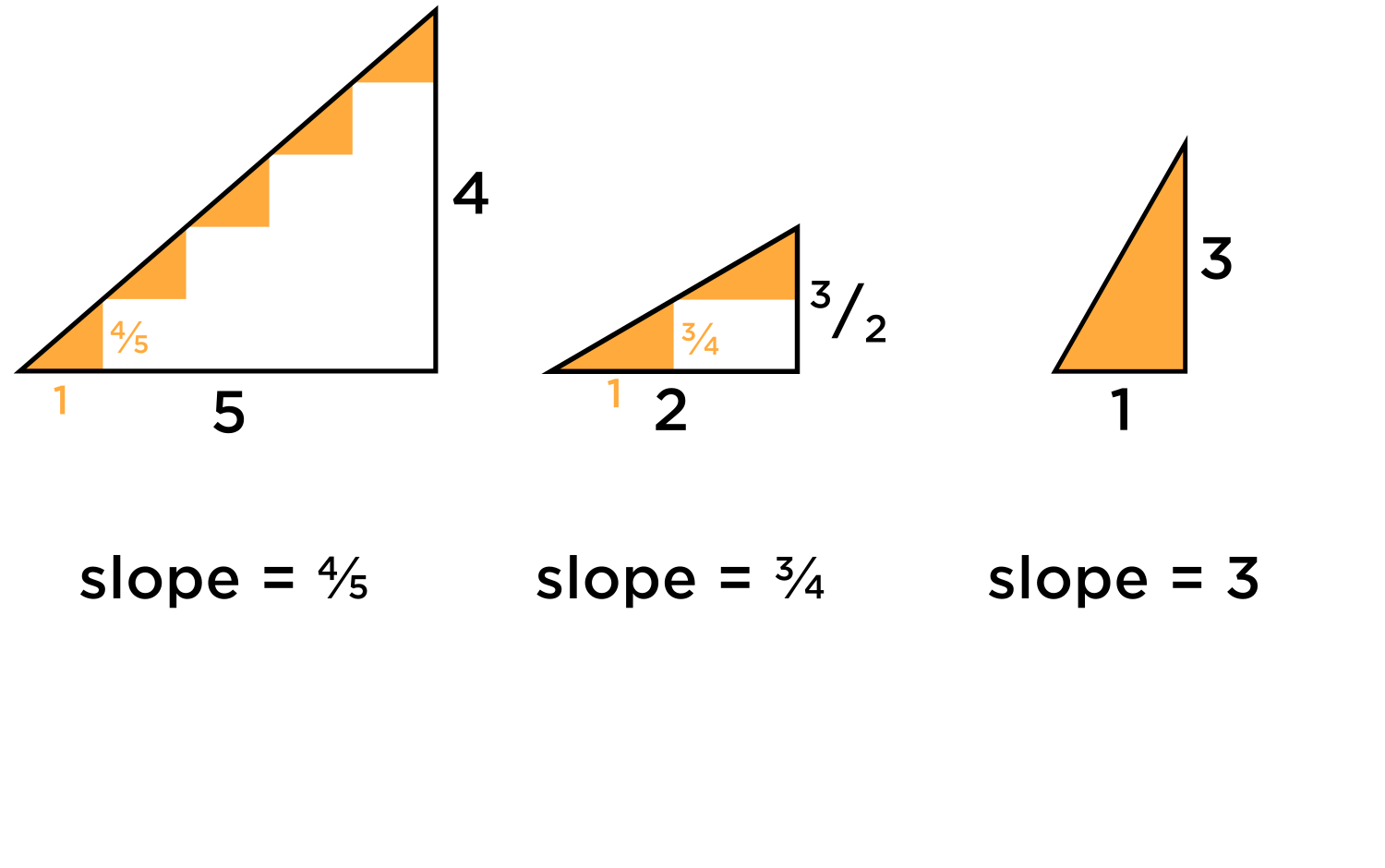# Meaning of Slope

• Someone asked this question over email:

"I was hoping you could help me understand in a more intuitive way why the gradient of a straight line is the ratio of the rise over the run? I guess I am trying to understand why division represents how much the rise changes in relation to the run? Why does dividing the rise into the run tell you how much run and rise change in relation to each other? Is that relationship anything like when we divide 6 into 3 (for example with apples) and which normally talk about grouping? How is our normal understanding of division related to the understanding that ratios talk about the relationship of change between the top and bottom of a fraction?"

I personally think gradient and slope are under-appreciated concepts. Most students memorize the definition of slope along with the mechanics of how to calculate it, without going much deeper. There is an intuitive way to look at it. If you're hiking on a trail and you want to describe how steep the trail is, how would you describe it? If you just say something like, "It's a 1 mile-long hike, but it takes one hour to go up it," that might indicate that you've got a very steep trail. If you say, "It's a trail that goes up to the top of a mountain which rises 100 ft above ground level," would that mean it's relatively flat, or relatively steep? The ambiguity of using length alone kind of begs us to find a different way to describe "steepness."

It's similar to how when describing the speed of a car, we don't just use distance. "My car went 60 miles," isn't very informative. We need to also know how much time it took to go the 60 miles. When shopping, looking at the sticker price alone doesn't tell you how cheap an item is. "This box of goldfish crackers is just $3!" We all know that it matters how big the box of goldfish crackers is. If you were to buy 3 pounds of goldfish crackers at$8, that would be cheaper than buying 1 pound of goldfish crackers costing $3. Unit cost, speed, and steepness are all quantities that fall into the category of "rate." These are all characteristics for which only one unit of measurement is insufficient to describe it. Rather, we need two measurements: Speed : Distance / Time Unit cost: $$/ Pound Steepness: Change in Height / Change in X direction These aren't just rates, they can be thought of as unit rates. Speed: miles traveled per 1 hour (1 hour is the standard unit of time) Unit cost:$$$ / 1 pound (1 pound is the standard unit of weight)
Steepness: Change in height / change in x direction (1 unit of change in x is the standard unit of slope)

It's easier when we use the same units for all of our rates, for the sake of comparison with other numbers. For example, which is cheaper, a box of goldfish crackers costing 10 pesos and weighing 11 ounces, or a box of goldfish crackers costing 400 cents and weighing 50 grams? If we use standard units, like  / pound, then comparing numbers is straightforward: you simply look at the number to see which is bigger.

Speed: 25 miles per hour vs. 15 miles per hour
Cost: $5 per pound vs.$4 per pound
Steepness: 2 vs. 1.5

A hill with a slope of 2 is steeper than a hill with a slope of 1.5. Easy!For slope, we can visualize the steepness another way: instead of a hill, let's pretend we're climbing a staircase. The width of each stair (this determines how much space your foot has when you step on the step) is set to be a standard length of 1. (You can use cm, ft, inches, etc., as long as it's consistent with your other measurements). The height of one single step is equal to the slope. If the slope number is bigger, then each step is taller, and the staircase is more steep. If the slope number is smaller, then each step is more shallow, and the staircase is less steep.

Here I've drawn some examples of upward-sloping lines. As you can see, the slope for the first two was calculated incorrectly. In the first case, only the total height (not the rate of height change per unit change in x) was used. In the second example, only the change in x was used. The third triangle is always a unit triangle, as its "change in x", or width, is already 1.We can turn each slanted line into a staircase where each step has a width of 1. The height of a single step is the value of the slope for each slanted line.https://forum.poshenloh.com/topic/395/in-the-equation-y-ax-b-which-coefficient-is-the-slope?_=1601899503208• @debbie
I still remember epols...• @RZ923 Yes!!$$\text{epols} = \frac{\text{change in } x}{\text{change in } y}$$